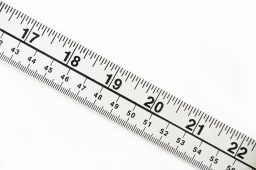# Half of 2

Half of Ethan’s string is equal to 2/3 of Kayla’s string. The total length of their strings is 10 feet.

How much longer is Ethan’s string than Kayla’s?

ftDid you find an error or inaccuracy? Feel free to write us. Thank you!Tips to related online calculators
Need help to calculate sum, simplify or multiply fractions? Try our fraction calculator.
Do you have a linear equation or system of equations and looking for its solution? Or do you have a quadratic equation?
Do you want to convert length units?